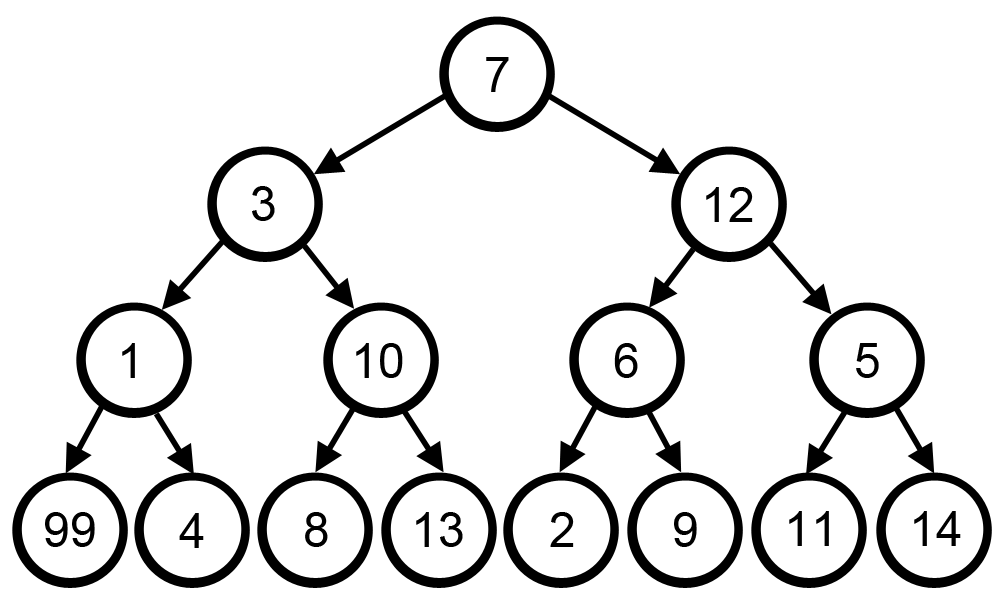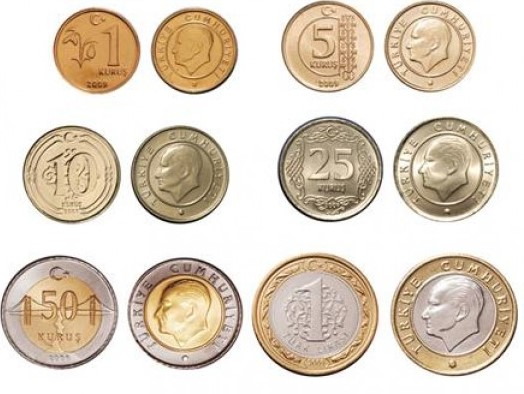# Greedy Algorithms

A greedy algorithm, as the name suggests, always makes the choice that seems to be the best at that moment. This means that it makes a locally-optimal choice in the hope that this choice will lead to a globally-optimal solution.

They never look backwards at what they’ve done to see if they could optimise globally. This is the main difference between Greedy and Dynamic Programming.

## Limitations of Greedy Algorithms

Sometimes greedy algorithm fails to find the optimal solution because it does not consider all available data and make choices which seems best at that moment.

A famous example for this limitation is searching the largest path in a tree.The greedy algorithm fails to solve this problem because it makes decisions purely based on what the best answer at the time is: at each step it did choose the largest number and solve the problem as 7 -> 12 -> 6 -> 9. Total is: 34.

But obviously, this is not the optimal solution. Correct solution to this problem is, 7 -> 3 -> 1 -> 99. Total is: 110.

## Minimum Coin Change Problem

A good example to understand Greedy Algorithms better is; the minimum coin change problem.

In this problem, the aim is to find the minimum number of coins with particular value which add up to a given amount of money. These types of optimization problems is often solved by Dynamic Programming or Greedy Algorithms.

Say you’re a cashier in Istanbul and need to give someone 2 lira (₺) and 67 kuruş (kr) using as few coins1 as possible. How would you do it?

For reference, this is the denomination of each coin in Turkey:

``````[1 kr, 5 kr, 10 kr, 25 kr, 50 kr, ₺1 (100 kr)]
``````For returning 2 lira (₺) and 67 kuruş (kr), you’d take the highest-value coin you could. A lira, another lira, then a 50 kr, a 10 kr, a 5 kr and finally two 1 kr. That’s a greedy algorithm, because you’re always greedily choosing the coin that covers the biggest portion of the remaining amount.

### Implementation

``````denominations = [1, 5, 10, 25, 50, 100]
# 100kr is ₺1

def return_change(change, denominations):
to_give_back =  * len(denominations)

# starting with the largest coin, goes through denominations list
# and also keeps track of the counter, pos.
for pos, coin in enumerate(reversed(denominations)):
# while we can still use coin, use it until we can't
while coin <= change:
change = change - coin
to_give_back[pos] += 1